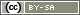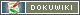This translation is older than the original page and might be outdated. See what has changed.

# Randomization: Questions

In some cases you need to randomize full questions. Therefore you have to use PHP-code. The integration of the PHP-code is the second step, so please read the chapter Randomization with PHP-Code first. The creation of the Random Generator and where to place it with the PHP-code in the questionaire is explainede in this chapter.

If the stimulus is a question you have to use the PHP-functions question() and value(). The first one is to define, which question should be displayed. value() is used to read out the value of the random generator (= 1, 2 or 3?). The code may look like this:

```if (value('RG01') == 2) {
question('AB01');
} elseif (value('RG01') == 3) {
question('AB02');
} else {
// This else-part could be deleted
// in this part nothing happens
}```

The value for the random generator (RG01) would display nothing, because the attendee is assigned to the controll group. In case the random generator took the value RG01=2, the attendee is shown the question AB01. And in the last case (RG01=3) the attendee gets the question AB02. Both the question-ID for the random generator and the question-IDs of the randomized questions have to be changed individually.

## Stimulus and Question together

It is also possible to randomize a question and a stimulus (image, text…) together. This is the case when you also want to query different questions depending on the stimulus.

```\$number = value('RG01');

if (\$number == 1) {
text('stimulusA');
question('AB01');
} elseif (\$number == 2) {
text('stimulusB');
question('AB01');
} elseif (\$number == 3) {
text('stimulusC');
question('AB02');
} elseif (\$number == 4) {
text('stimulusD');
question('AB02');
}```

In this example the stimulus A gets another question displayed than the other groups.

Note: If the question to the stimulus stayes the same you do not need to indicade it by hard. In this case it is enough to place the question under the stimulus or on the next page undepending to the question.

## Stimulus and Question on different Pages

If the stimulus and the (respectively associated) question are to be shown on different pages, a filter is installed on both pages. Once the random number has been drawn, it is available (in the example as variable `RG01`) on all following pages. So you need to place the random number generator (or the urn draw) only once in the questionnaire, usually right before the first filter.

```// PHP-code on the page with the stimulus
\$number = value('RG01');

if (\$zahl == 1) {
text('stimulusA');
} elseif (\$number == 2) {
text('stimulusB');
} elseif (\$number == 3) {
text('stimulusC');
} elseif (\$number == 4) {
text('stimulusD');
}```
```// PHP-code on the page with the question
\$number = value('RG01');

if (\$number == 1) {
question('AB01');
} elseif (\$number == 2) {
question('AB01');
} elseif (\$number == 3) {
question('AB02');
} elseif (\$number == 4) {
question('AB02');
}```

Note: Of course, this solution is not necessary if the same question is always displayed regardless of the stimulus.

Tip: This variable can be used to distribute related stimuli (e.g. page 1 to 3 of a newspaper article) over several pages.

## Questions on different Pages

If you want the questions to appear on different pages in the questionnaire, you have to filter in two places:

```//Place on the first page
\$number = value('RG01');  // reading out the random number of RG01

// display one or the other question
if (\$number == 1) {
question('AB01');
} else {
question('AB03');
}```
```//Place on the second page
\$number = value('RG01');
if (\$number == 1) {
question('AB02');
} else {
question('AB004');
}```

In the example, by chance, either question AB01 is displayed first and then AB02 later – or AB03 first and then AB04.

Note: In this way it is also possible to create different groups of respondents to whom the same questions are to be presented only in different but fixed orders.

Note: If you want to randomize whole pages, this approach can quickly become confusing. Therefore read the chapter Randomization: Pages!